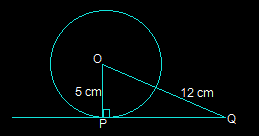Guru

# A tangent PQ at a point P of a circle of radius 5 cm meets a line through the centre O at a point Q so that OQ = 12 cm. Length PQ is : (A) 12 cm (B) 13 cm (C) 8.5 cm (D) √119 cm Q.3

• 0

What is the best way to solve this question of class 10th ncert of exercise 10.1 of circles chapter of question no.3, please help me to solve this question , it is an important question for class 10Th A tangent PQ at a point P of a circle of radius 5 cm meets a line through the centre O at a point Q so that OQ = 12 cm. Length PQ is : (A) 12 cm (B) 13 cm (C) 8.5 cm (D) √119 cm

Share

1. This answer was edited.In the above figure, the line that is drawn from the centre of the given circle to the tangent PQ is perpendicular to PQ.

And so, OP ⊥ PQ

Using Pythagoras theorem in triangle ΔOPQ we get,

OQ2 = OP2+PQ2

(12)2 = 52+PQ2

PQ2 = 144-25

PQ2 = 119

PQ = √119 cm

So, option D i.e. √119 cm is the length of PQ.

• 0